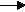gcsescience.com                                       20                                       gcsescience.com

Moles

Volumes of Gases - Avogadro's Law.

Avogadro's Law says that the same volume of gas
contains
the same number of particles.
It does not matter what gas you have, they all contain
the same number of particles provided
that they are at the same temperature and pressure.

This leads on to an even more useful fact.
At room temperature (25 °C) and
one atmosphere pressure (101kPa)
one mole of any gas occupies a volume of 24,000 cm3.
24,000 cm3 is the same volume as 24 litres.

The equation which converts moles into volume is

volume = moles x 24,000 cm3

Example 1
.

What volume of carbon dioxide
is produced by burning 6 g  of carbon in air?

Method.
1) Find how many moles of carbon are present in 6 g  of carbon.
RAM of C = 12.

moles = mass ÷ RAM

moles = 6 ÷ 12

= 0·5 moles of carbon.

2) Write the equation for the reaction.

carbon + oxygencarbon dioxide.
C(s)  +    O2(g)CO2(g)

Use the big numbers to find the proportion of reactant to product.
1C gives 1CO2, the proportion is 1 to 1,
so 0·5 moles of carbon will make 0·5 moles of carbon dioxide.

3) Convert moles into volume.

volume moles x 24,000 cm3

volume 0·5 x 24,000 cm3
= 12,000 cm3 of carbon dioxide.

So, burning 6 g  of carbon in air
will produce 12,000 cm3 of carbon dioxide.

gcsescience.com       The Periodic Table       Index       Moles Quiz       gcsescience.com